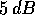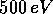Next: 1 Jan/9516:21 - Up: Morphology during a Complete Previous: Morphology during a Complete

## SuperDARN Maps of Double-peaked Doppler Velocity Spectra

The following presentation of experimental results consists of a series of graphs for each time period considered in an attempt to describe the situation in as much detail as possible from the limited data available. There are nine events shown. Each will be discussed in detail in the following sections using a sequence of six plots.

The first plot in the sequence is an overview map indicating the region where ACFs were measured with the Saskatoon and Kapuskasing radars. Only ACFs with a signal to noise ratio greater thanare considered. The ranges where double peaked spectra were observed are shown as solid points in these maps. The second plot shows the locations of the double-peaked spectra and the satellite flight path. This graph also shows the satellite track mapped to the conjugate positions at F- and E-layer heights and the time (in seconds of the day) along the flight path. This time is the link between the geographic radar plot and the plots that are generated from satellite data which use the time as the x coordinate. The third plot for each event shows the average energy and the energy flux of the electrons. It is important to note that the energy integration is stopped atfor the correct use of the Robinson model and that the energy values at low fluxes are highly variable and not very accurate. In the case of the Robinson model, the characteristic energy is equal to the average energy since an exponential power law distribution of the formis assumed.The fourth graph in the sequence shows the ionization rates of the E-layer (at 100 km) and the F-layer (320 km) as derived from the complete electron spectrum by modeling. This graph is very useful in determining changes of the ionosphere at F-layer heights due to soft electron precipitation. Such changes at low energy do not show clearly in the plot of average energy. In the fifth graph, the estimated Pedersen and Hall conductivities for the E-layer are shown, as directly derived from the energy and flux data. The spatial gradient of the Pedersen conductance is calculated and mentioned, if relevant, but not shown. One should note that the conductivity values are accurate only if the flux is sufficiently high. Each discussion will conclude with a sixth plot giving a general convection velocity map derived from the Doppler velocities of both radars over a period of 15 minutes (centered at the time of the event); this is a standard SuperDARN data product.Next: 1 Jan/9516:21 - Up: Morphology during a Complete Previous: Morphology during a Complete

Andreas Schiffler
Wed Oct 9 10:05:17 CST 1996